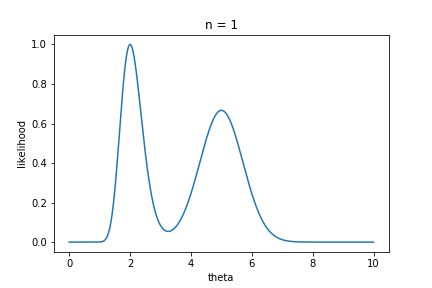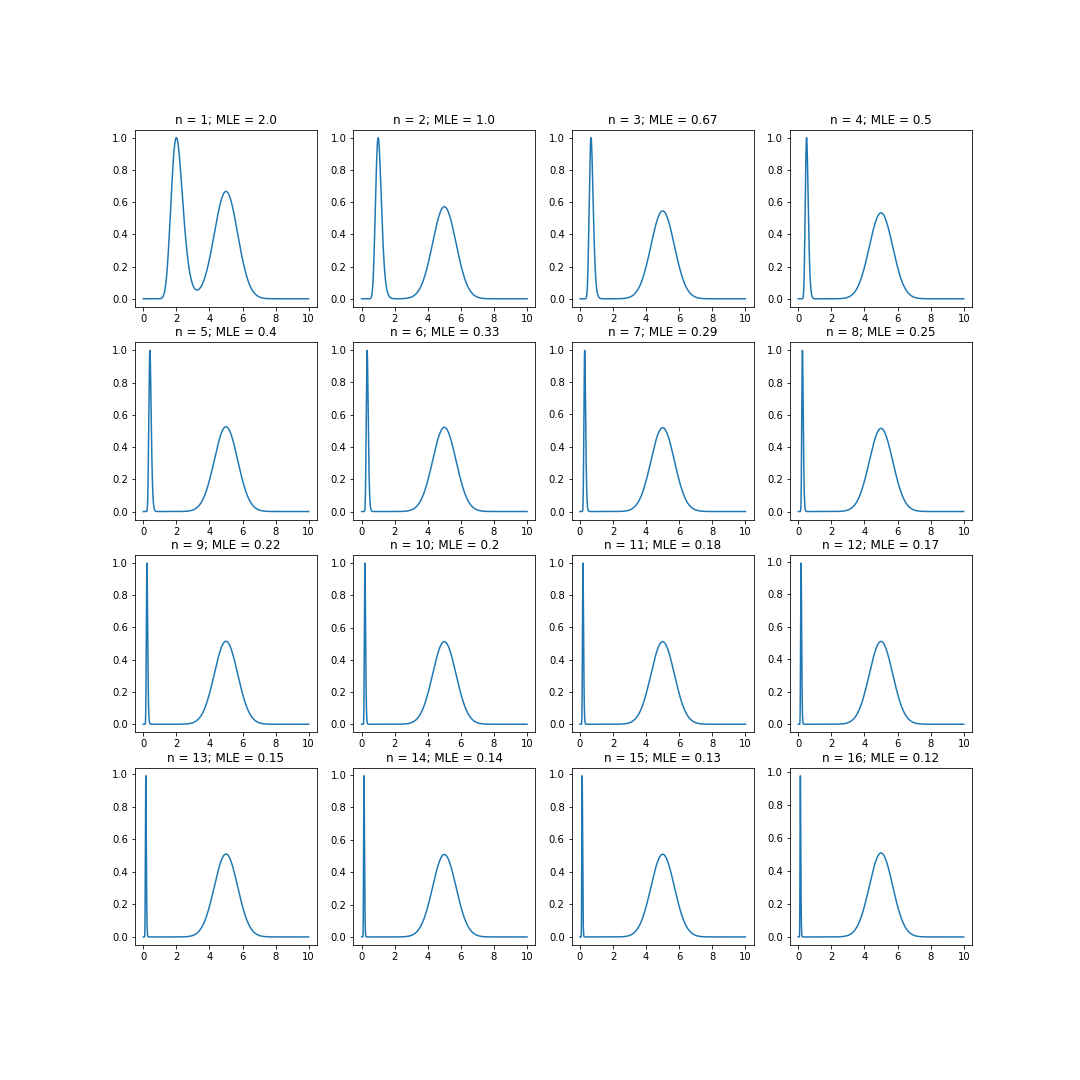# Consistency of MLE

Maximum likelihood estimation (MLE) is one of the most popular and well-studied methods for creating statistical estimators. This post will review conditions under which the MLE is consistent.

## MLE

Maximum likelihood estimation is a broad class of methods for estimating the parameters of a statistical model. In particular, as its name suggests, its main goal is to find the parameter values that make the data “most likely” under some assumed family of densities.

Let $X_1, \dots, X_n$ be some data that were generated from an assumed family of densities $f(X_i, \theta)$. The likelihood of the data $L$ for some value of $\theta$ is then just the product of the density evaluated at each of the samples:

$L = \prod\limits_{i = 1}^n f(X_i, \theta).$

Often the log-likelihood is more convenient to work with, so we’ll often write the log-likelihood $\ell_n$ as

$\ell_n(\theta) = \log \left(\prod\limits_{i = 1}^n f(X_i, \theta).\right) = \sum\limits_{i = 1}^n \log f(X_i, \theta).$

Assume that the data were generated under some true parameter $\theta_0$. Then, the goal of estimation is to use the data to find a guess of the parameter value, $\hat{\theta}$, that is close to $\theta_0$.

The MLE finds the value $\hat{\theta}$ such that:

$\hat{\theta}_n = \text{arg}\max_{\theta \in \Theta} \ell_n(\theta).$

## Consistency

An estimator $\hat{\theta}$ is consistent if it approaches the true value $\theta_0$ as more data is observed. In particular, a consistent estimator $\hat{\theta}$ converges in probability to $\theta_0$. That is, for all $\epsilon > 0$,

$\lim_{n \to \infty} \mathbb{P} \left[ |\hat{\theta}_n - \theta_0| > \epsilon \right] = 0.$

## Consistency of MLE

The MLE is consistent when particular conditions are met:

1. The model must be identifiable.
2. The parameter space $\Theta$ must be compact.
3. The density function must be continuous.
4. The log-likelihood must converge uniformly:
$\sup_{\theta \in \Theta} ||\ell_n(\theta) - \ell(\theta)|| \to_p 0$

where $\ell(\theta)$ is the expected log-likelihood, $\ell(\theta) = \mathbb{E}[\log f(X_i, \theta)].$

## Uniform convergence condition

Let’s dig a bit deeper on condition (4). Recall that our MLE estimator $\hat{\theta}_n$ is taking the maximum of a function that is based on $n$ samples. As $n$ increases, the function changes, and so does the maximum value. In order to show consistency, we need to keep track of the limiting behavior of both the function and its maximum.

The primary reason that this condition is necessary is so that the maximum of the limiting function and the limit of the maximum of these functions are the same.

This jargon can be pretty confusing, so let’s look at an example (credit to Prof. Matias Cattaneo for this example; see references) where this uniform convergence breaks down.

Assume we have a likelihood function that looks like the plot below, where the true parameter value $\theta_0 = 5$. This is the likelihood function for $1$ sample. Already, we can tell that the MLE will be incorrect in this example: there are two peaks, and the one around $\hat{\theta} = 2$ is higher.Now, notice what happens to the shape of the likelihood function as $n$ becomes larger.The left peak gets closer and closer to $0$, and eventually (although you can’t see it here) it gets arbitrarily close to $0$. That is, it essentially disappears in the limit.

Let’s think about the two quantities we want to measure here: maximum of the limiting function and the limit of the maximum of these functions.

The limiting function will simply be a peak around $\hat{\theta} = 5$, since the left peak disappears in the limit.

However, the limit of the maximum of these functions is at $0$ because the maximum is always approaching $0$. So the limit of the maximum likelihood estimator as $n \to \infty$ is $0$, but recall that the true paramter $\theta_0 = 5$.

Thus, in this case, we can see that the log-likelihood will not converge uniformly to the expected log-likelihood, and the MLE will not be consistent.

## Conclusion

Here we’ve reviewed some of the sufficient conditions for the MLE to be a consistent estimator. To build intuition, we saw one pathological case where the likelihood function does not converge uniformly to its expectation.